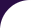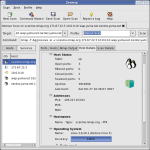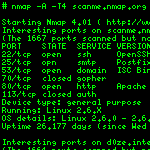Intro Reference Guide Book Install Guide Download Changelog Zenmap GUI Docs Bug Reports OS Detection Propaganda Related Projects In the Movies In the News# Library `unittest`

Unit testing support for NSE libraries.

This library will import all NSE libraries looking for a global variable `test_suite`. This must be a callable that returns true or false and the number of tests that failed. For convenience, the `unittest.TestSuite` class has this property, and tests can be added with `add_test`. Example:

```local data = {"foo", "bar", "baz"}
test_suite = unittest.TestSuite:new()
test_suite:add_test(equal(data, "bar"), "data should equal 'bar'")```

The library is driven by the unittest NSE script.

## Functions

 equal (a, b) Test for equality expected_failure (test) Expected failure test identical (a, b) Test two values for equality, recursively if necessary. is_false (value) Test for falsehood is_nil (value) Test for nil is_true (value) Test for truth keys_equal (a, b) Test associative tables for equality, 1 level deep length_is (t, l) Test length lt (a, b) Test less than lte (a, b) Test less than or equal to make_test (test, fmt) Test creation helper function. Turns a simple function into a test factory. not_equal (a, b) Test for inequality not_nil (value) Test for not nil run_tests (to_test) Run tests provided by NSE libraries table_equal (a, b) Test tables for equality, 1 level deep testing () Check whether tests are being run TestSuite.__call (self) Run tests. Runs all tests in the TestSuite, and returns the number of failures. TestSuite.add_test (self, test, description) Add a test. TestSuite.new (self) Creates a new TestSuite object TestSuite.setup (self) Set up test environment. Override this. TestSuite.teardown (self) Tear down test environment. Override this.

## Functions

equal (a, b)

Test for equality

### Parameters

• a: The first value to test
• b: The second value to test

### Return value:

bool True if a == b, false otherwise.
expected_failure (test)

Expected failure test

### Parameters

• test: The test to run

### Return value:

function A test for expected failure of the test
identical (a, b)

Test two values for equality, recursively if necessary.

This function checks that both values are indistinguishable in all but memory location.

### Parameters

• a: The first value to test.
• b: The second value to test

### Return values:

1. bool True if values are indistinguishable, false otherwise.
2. note Nil if values are indistinguishable, description of distinguishability otherwise.
is_false (value)

Test for falsehood

### Parameters

• value: The value to test

### Return value:

bool True if value is a boolean and false
is_nil (value)

Test for nil

### Parameters

• value: The value to test

### Return value:

bool True if the value is nil, false otherwise.
is_true (value)

Test for truth

### Parameters

• value: The value to test

### Return value:

bool True if value is a boolean and true
keys_equal (a, b)

Test associative tables for equality, 1 level deep

### Parameters

• a: The first table to test
• b: The second table to test

### Return value:

bool True if a[k] == b[k] for all k in a and b
length_is (t, l)

Test length

### Parameters

• t: The table to test
• l: The length to test

### Return value:

bool True if the length of t is l
lt (a, b)

Test less than

### Parameters

• a: The first value to test
• b: The second value to test

### Return value:

bool True if a < b, false otherwise.
lte (a, b)

Test less than or equal to

### Parameters

• a: The first value to test
• b: The second value to test

### Return value:

bool True if a <= b, false otherwise.
make_test (test, fmt)

Test creation helper function. Turns a simple function into a test factory.

### Parameters

• test: A function that returns true or false depending on test
• fmt: A format string describing the failure condition using the arguments to the test function

### Return value:

function that generates tests suitable for use in add_test
not_equal (a, b)

Test for inequality

### Parameters

• a: The first value to test
• b: The second value to test

### Return value:

bool True if a != b, false otherwise.
not_nil (value)

Test for not nil

### Parameters

• value: The value to test

### Return value:

bool True if the value is not nil, false otherwise.
run_tests (to_test)

Run tests provided by NSE libraries

### Parameters

• to_test: A list (table) of libraries to test. If none is provided, all libraries are tested.
table_equal (a, b)

Test tables for equality, 1 level deep

### Parameters

• a: The first table to test
• b: The second table to test

### Return value:

bool True if #a == #b and a[i] == b[i] for every i<#a, false otherwise.
testing ()

Check whether tests are being run

Libraries can use this function to avoid the overhead of creating tests if the user hasn't chosen to run them. Unittesting is turned on with the `unittest.run` script-arg.

### Return value:

true if unittests are being run, false otherwise.
TestSuite.__call (self)

Run tests. Runs all tests in the TestSuite, and returns the number of failures.

• self:

### Return values:

1. failures The number of tests that failed
2. tests The number of tests run

### Parameters

• self:
• test: Function that will be called with the TestSuite object as its only parameter.
• description: A description of the test being run
TestSuite.new (self)

Creates a new TestSuite object

• self:

### Return value:

TestSuite object
TestSuite.setup (self)

Set up test environment. Override this.

### Parameters

• self:
TestSuite.teardown (self)

Tear down test environment. Override this.

• self: